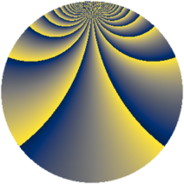# Properties

 Label 1470.2.bgLevel $1470$ Weight $2$ Character orbit 1470.bg Rep. character $\chi_{1470}(121,\cdot)$ Character field $\Q(\zeta_{21})$ Dimension $432$ Sturm bound $672$

# Related objects

## Defining parameters

 Level: $$N$$ $$=$$ $$1470 = 2 \cdot 3 \cdot 5 \cdot 7^{2}$$ Weight: $$k$$ $$=$$ $$2$$ Character orbit: $$[\chi]$$ $$=$$ 1470.bg (of order $$21$$ and degree $$12$$) Character conductor: $$\operatorname{cond}(\chi)$$ $$=$$ $$49$$ Character field: $$\Q(\zeta_{21})$$ Sturm bound: $$672$$

## Dimensions

The following table gives the dimensions of various subspaces of $$M_{2}(1470, [\chi])$$.

Total New Old
Modular forms 4128 432 3696
Cusp forms 3936 432 3504
Eisenstein series 192 0 192

## Trace form

 $$432q + 36q^{4} + 36q^{9} + O(q^{10})$$ $$432q + 36q^{4} + 36q^{9} + 4q^{10} - 4q^{11} - 16q^{13} + 12q^{14} + 36q^{16} - 8q^{17} - 4q^{19} + 16q^{22} + 36q^{25} - 32q^{26} + 32q^{29} - 8q^{31} + 8q^{33} + 16q^{34} - 16q^{35} - 72q^{36} - 48q^{37} + 104q^{38} - 40q^{39} + 4q^{40} + 88q^{41} + 96q^{42} - 32q^{43} + 52q^{44} - 4q^{46} + 208q^{47} + 104q^{49} + 104q^{51} + 8q^{52} + 200q^{53} + 16q^{55} + 56q^{56} - 32q^{57} + 104q^{58} + 116q^{59} + 8q^{61} + 80q^{62} - 72q^{64} - 4q^{65} - 8q^{68} + 32q^{69} + 64q^{71} - 176q^{73} - 48q^{74} + 8q^{76} - 144q^{77} - 16q^{79} + 36q^{81} + 16q^{82} - 184q^{83} + 16q^{85} - 16q^{86} - 64q^{88} + 64q^{89} - 8q^{90} + 164q^{91} - 40q^{94} - 16q^{95} + 288q^{97} + 8q^{99} + O(q^{100})$$

## Decomposition of $$S_{2}^{\mathrm{new}}(1470, [\chi])$$ into newform subspaces

The newforms in this space have not yet been added to the LMFDB.

## Decomposition of $$S_{2}^{\mathrm{old}}(1470, [\chi])$$ into lower level spaces

$$S_{2}^{\mathrm{old}}(1470, [\chi]) \cong$$ $$S_{2}^{\mathrm{new}}(49, [\chi])$$$$^{\oplus 8}$$$$\oplus$$$$S_{2}^{\mathrm{new}}(98, [\chi])$$$$^{\oplus 4}$$$$\oplus$$$$S_{2}^{\mathrm{new}}(147, [\chi])$$$$^{\oplus 4}$$$$\oplus$$$$S_{2}^{\mathrm{new}}(245, [\chi])$$$$^{\oplus 4}$$$$\oplus$$$$S_{2}^{\mathrm{new}}(294, [\chi])$$$$^{\oplus 2}$$$$\oplus$$$$S_{2}^{\mathrm{new}}(490, [\chi])$$$$^{\oplus 2}$$$$\oplus$$$$S_{2}^{\mathrm{new}}(735, [\chi])$$$$^{\oplus 2}$$